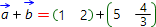Vectors addition can be accomplished in several different ways, depending on their representation form. If vectors represented by coordinates, their sum is also the vector with coordinates which are the sum of corresponding coordinates of initial vectors. If vectors are given in geometrical form, to find their sum one can use parallelogram rule, which shown on the picture below.From the picture it follows, that one need to match the initial points of vectors being summed, and on their final points - complete corresponding sides of parallelogram. The sum of the vectors is the vector which is the parallelogram's diagonal.

Our calculator can find step by step solution for the sum of vectors in coordinate form. As vector's coordinates, one can use coordinates of initial and final points of vector. To find vectors sum one should choose dimension, representation form and input vectors coordinates. As coordinates of vector one can use not only numbers (1, 5 ect.) and fractions (1/2, 5/8 ect.), but also the parameters (x, y ect.)by:by:= { }= { }